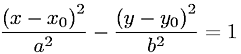Equations > Geometry > Curves and Shapes > Equation of a Hyperbola

### Equation of a HyperbolaLatex Code:

MathML Code:

 $\frac{\left(x-{x}_{0}\right)}^{2}{a}^{2}-\frac{\left(y-{y}_{0}\right)}^{2}{b}^{2}=1$

MathType 5.0: